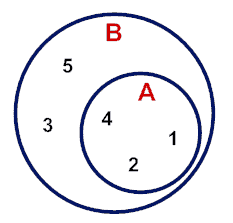# Python Set issuperset: Complete Overview

In Python, there are two different approaches of checking whether the given pair of sets are in the superset relationship or not; they are as follows:

1. issuperset Method
2. >= Operator

## Python Set issuperset()

The Set issuperset() is a built-in Python function that returns True if a set has every element of another Set (passed as an argument). If not, it returns False. The Set A is said to be the Superset of set B if all elements of B are in A.

Before learning the Python issuperset() method, we should be clear with the Superset. If all the values of one set are present in another set, then the second set is called Superset of the first set.

Let’s understand the difference between subset and Superset with an example:Consider this image, there are two sets, A={1,2,4} and B={1,2,3,4,5}. So we can see that all the elements of set A are present in set B. So here, B is called Superset of set A. Also, set A is called the Subset of set B.

The python set issuperset() method helps us to find if a set is a superset of another set or not.

The issuperset() Method takes iterable (list, tuple, dictionary, set, and string) as an argument; if the iterable other than set is passed, it first converts an iterable object to the set object and then checks whether all items of a set ( passed as an argument ) are also present in the set ( on which issuperset method is invoked ).

If yes, then it returns True; otherwise, False.

### Syntax

```First_Set.issuperset(Second_Set)
```

### Arguments

Here, this syntax will check if First_Set is a superset of Second_Set or not.

### Return Value

The issuperset() function returns two types of values:

True: If First_Set is a superset of Second_Set

False: If First_Set is not a superset of Second_Set

See the following code.

```# app.py

# Declaring two sets
# Even nums between 2 and 10
set1 = {2, 4, 6, 8, 10}
# Multiple of 4 from 4 to 10
set2 = {4, 8}
# Multiple of 4 from 4 to 20
set3 = {4, 8, 12, 16, 20}

# priting both the sets
print("Set1 is: ", set1)
print("Set2 is : ", set2)
print("Set3 is: ", set3)
# Now we will find if they are disjoint
print("Is Set1 Superset of Set2? ", set1.issuperset(set2))
print("Is Set1 Superset of Set3? ", set1.issuperset(set3))
```

#### Output

```Set1 is:  {2, 4, 6, 8, 10}
Set2 is :  {8, 4}
Set3 is:  {4, 8, 12, 16, 20}
Is Set1 Superset of Set2?  True
Is Set1 Superset of Set3?  False
```

In this program, we have declared three sets, Set1, which is even numbers from 1 to 10, Set2, which contains a multiple of 4 from 4 to 10; and Set3, which includes a multiple of 4 from 4 to 20.

Now, we are finding if Set1 is a superset of Set2 or not. So, we can see that all the elements of Set2 are present in Set1. So, Set1 is a superset of Set2.

On the other side, we can see that not all the elements are present in Set1 of Set3, so Set1 is not a superset of Set3. That’s why the return value is False.

That’s it for this tutorial.

Python set issubset()

Python set isdisjoint()

Python set intersection_update()

Python set intersection()